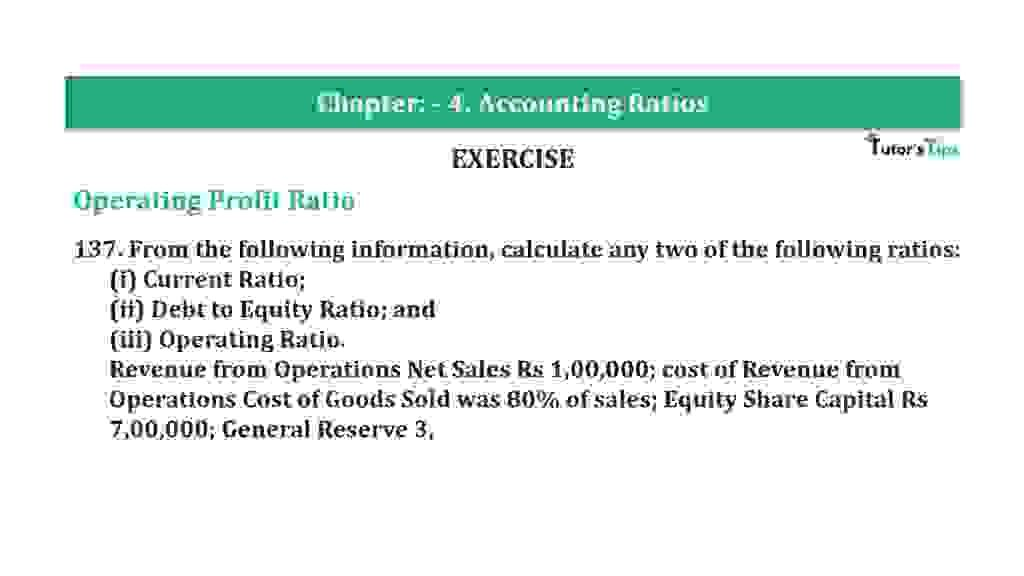# Question 137 Chapter 4 of +2-B – T.S. Grewal 12 ClassQuestion 137 Chapter 4 of +2-B

Miscellaneous

137. From the following information, calculate any two of the following ratios:
(i) Current Ratio;
(ii) Debt to Equity Ratio; and
(iii) Operating Ratio.
Revenue from Operations Net Sales Rs 1,00,000; cost of Revenue from Operations Cost of Goods Sold was 80% of sales; Equity Share Capital Rs 7,00,000; General Reserve 3.

### The solution of Question 137 Chapter 4 of +2-B: –

I

 Current Assets = Quick Assets + Closing Stock + Prepaid Expenses = Rs 6,00000 + Rs 50,000 + Rs 10,000 = Rs. 6,60,000

 Current Ratio = Current Assets Current Liabilities
 Current Ratio = Rs. 6,60,000 Rs. 4,00,000 = 1.65 : 1

II

 Long-term Debts = Rs 5,00,000 Shareholder’s Funds = Equity Share Capital + General Reserve = Rs 7,00,000 − Rs 3,00,000 = Rs 10,00,000
 Average Inventory = Long-term Debts Equity
 = Rs 5,00,000 Rs 10,00,000 = 0.5 : 1

III

 Sales = Rs 1,00,000 Cost of Goods Sold = Rs 80,000 Operating Expenses = Rs 10,000 = Cost of Goods Sold + Operating Expenses = Rs 80,000 + Rs 10,000 = Rs 90,000
 Operating Ratio = Operating Cost X 100 Net Sales
 Operating Ratio = Rs 90,000 X 100 Rs 1,00,000 = 90%

Balance Sheet: Meaning, Format & Examples

Thanks, Please Like and share with your friends

Comment if you have any question.

Also, Check out the solved question of previous Chapters: –

### T.S. Grewal’s Double Entry Book Keeping (Vol. II: Accounting for Companies)T.S. Grewal’s Analysis of Financial Statements